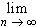#Interactive Real Analysis

Next | Previous | Glossary | Map

## 8.1. Pointwise Convergence

### Proposition 8.1.6: Pointwise Convergence defines Function

If { fn(x) } converges pointwise, thenfn(x) = f(x) is a well-defined function.

There isn't much to prove. We know that since fn converges pointwise, it converges for each fixed x to a limit L(x). The function defined via

f(x) = L(x)

is indeed a function because ... well, because ... let's see, to be a true function we would have for each x exactly one f(x) = L(x). But that must be true by some elementary property of numeric sequences.

Which one?Next | Previous | Glossary | Map